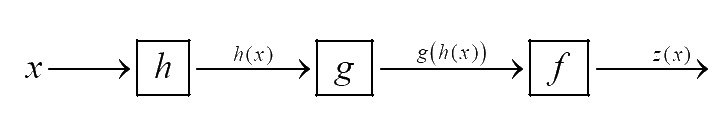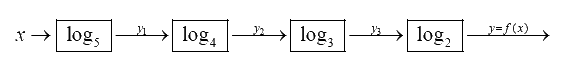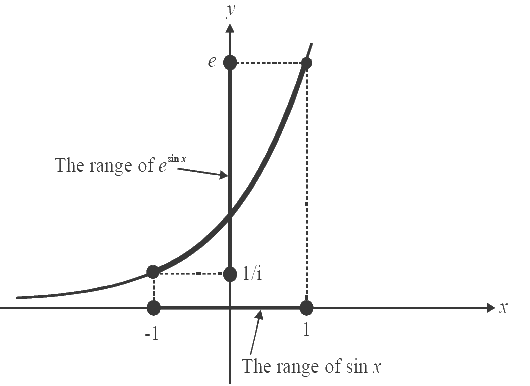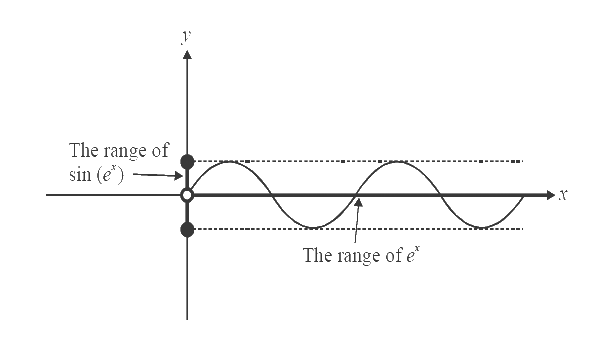# Examples on Exponential and Logarithmic Functions Set 2

Go back to  'Functions'

Example- 28

Find the domains for the function.

$f(x) = \sin \left( {\ln \left( {\frac{{\sqrt {4 - {x^2}} }}{{1 - x}}} \right)} \right)$

Solution: This is an example of a composition of functions.

$f\left( {g\left( {h..........(x)} \right)} \right)$

The previous question was a composition of four ‘log’ functions

Suppose we have a composite function $$z(x) = f\left( {g\left( {h(x)} \right)} \right)$$. Its is helpful to visualise z(x) in the following manner:To determine the domain of $$z(x),$$ it is intutive that we start from the rightmost side (or the outermost function, $$f$$ ) and analyses what values $$g(h(x))$$ (the domain for $$f$$ ) can take; and then accordingly what values $$h(x)\ {the domain for \(g$$} can take, and finally, what values $$x$$ can take.

Visualizing the example of the previous question in this manner, we getWe require $${y_3} > {\rm{ }}0$$ (the domain for the outermost function, log2)

This gives $${y_2} > {\rm{ }}1{\rm{ }}$$ (the domain for log3) and so on.

Applying this concept to our current example, we see that the domain for the ‘sin’ function is $$\mathbb{R}$$

Therefore, $$\ln \left( {\frac{{\sqrt {4 - {x^2}} }}{{1 - x}}} \right) \in \,\mathbb{R}$$

Now, the domain for the ‘ln’ function is $${\mathbb{R}^ + }$$ (positive real numbers)

Hence we require \begin{align}\frac{{\sqrt {4 - {x^2}} }}{{1 - x}} > 0\end{align}

The numerator is defined for $$x \in [ - 2,\;2],$$ the denominator for $$x \ne 1.$$ Also, the numerator is always positive (except when $$x=\pm 2,$$ when it becomes 0) (square root function yields non-negative output by definition)

The denominator is positive when $$x < 1.$$

Therefore, $$x$$ should satisfy the following conditions:

$x{\mkern 1mu} \in {\mkern 1mu} [ - 2,2]{\rm{ }}\quad and \quad {\rm{ x }} \ne 1{\rm{ }}\quad and \quad {\rm{ x }} \ne \pm 2{\rm{ }}\quad and \quad {\rm{ }}x < 1$

$\Rightarrow {\mkern 1mu} D = ( - 2,1)$

Example- 29

Find the domain and range of

(a) $$f(x) = {e^{\sin x}}$$                                    (b) $$f(x)x = \sin ({e^x})$$

(c) $$f(x) = \ln \,(\sin ({e^x}))$$                          (d) $$f(x) = \ln (\sin (\ln \,x))$$

Solution: (a) As $$x$$ varies over $$\mathbb{R}$$, $$sin\,x$$ varies in [–1, 1], so $${e^{\sin x}}$$ will vary from a minimum value of \begin{align}{e^{ - 1}} = \frac{1}{e}\end{align} to a maximum value of $${e^1} = e$$. Let us depict this graphically.Therefore,

$$\begin{gathered} D = \mathbb{R} \hfill \\ R = \left[ {\;\frac{1}{e},\;e\;} \right] \hfill \\ \end{gathered}$$

(b) As $$x$$ varies over $$\mathbb{R},\;{e^x}$$ varies in $$(0,\;\infty )$$. The range of $$\sin ({e^x})$$ will be the range of the sin function: [–1, 1]. Convince yourself about this:Therefore,

$$\begin{gathered}D = \mathbb{R} \hfill \\R = [ - 1,\;1] \hfill \\\end{gathered}$$

(c) In this case, we’ll have a constraint on the domain:

$$f(x) = l\,n\,\left( {\sin ({e^x})} \right)$$

$${\rm{}} \Rightarrow \quad \sin \left( {{e^x}} \right){\rm{}}must\;be\;positive\;for\;ln\;to\;be\;defined$$

$$\Rightarrow \qquad \sin \left( {{e^x}} \right) > 0$$

What values can $${e^x}$$ take then for $$\sin \left( {{e^x}} \right)$$ to be positive? Well, $${e^x}$$ can lie in $$(0,\;\pi ),\;(2\pi ,\;3\pi ),\;(4\pi ,\;5\pi ).......$$and generally in $$\left( {2n\pi ,\;(2n + 1)\pi } \right),\;n \ge 0$$. Look at the graph of $$sin\,x$$ and convince yourself that $$sin\,x$$ is positive in these intervals. {Intervals on the negative side have not been taken since $${e^x}$$ cannot be negative}. So,

If $${e^x} \in (0,\;\pi ) \qquad \Rightarrow \qquad x \in ( - \infty ,\;l\,n\;\pi )$$

If $${e^x} \in (2\pi ,\;3\pi ) \qquad \Rightarrow \qquad x \in (l\,n\;2\pi ,\;l\,n\;3\pi )$$

Generalizing, the set of allowed values for $$x,$$ i.e., the domain, is all intervals of the form

$$\left( {l\,n\;2n\pi ,\;l\,n(2n + 1)\pi } \right),\;\;\;n \ge 0,\;\;n \in \mathbb{Z}$$

What is the range? For the given domain $$\sin ({e^x})$$, will take positive values, but $$\sin ({e^x})$$ cannot rise above 1. Thus,

\begin{align}&\qquad\qquad\quad\sin ({e^x}) \in (0,\;1]\\&\Rightarrow \qquad \ln \,(\sin ({e^x})) \in (-\infty ,\;0]\end{align}

The range is $$( -\infty ,\;0]$$

(d) We are only providing the answers. The justification is left to the reader as an exercise:

D = All intervals of the form $$\left( {{e^{2n\pi }},\;{e^{(2n + 1)\pi }}} \right),\;n \in \mathbb{Z}$$

$$R = \left( { - \infty ,\;0} \right]$$

## TRY YOURSELF - III

Q.1 Find the domains and range of the following functions:

 (a) $$f(x) = {e^{\{ x\} }}$$ (b) $$f(x) = ln\,\left( {\sqrt x } \right)$$ (c) $$f(x) = {e^{[x]}}$$ (d) $$f(x) = {e^{\tan x}}$$ (e) $$f(x) = ln\left( {{x^2} + 1} \right)$$ (f) $$f(x) = ln\{ x\}$$